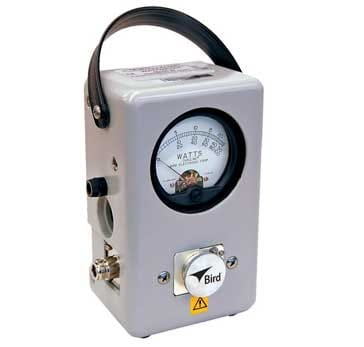# VSWR Formulas & Calculations

## There are many different equations or formulas that can be used to calculate and determine the VSWR that exists in a feeder.

VSWR & Transmission Line Theory Tutorial Includes:
What is VSWR?     Reflection Coefficient     VSWR formulas & calculations     How to measure VSWR     How to use a VSWR meter     Simple SWR bridge circuit     What is return loss     VSWR / Return Loss Table

There are many different formulas or equations that can be used to calculate VSWR from a variety of different parameters.

As VSWR is an important aspect associated, especially with feeders and antennas, it can be helpful to know the different relationships and formulas for calculating VSWR.

The normal way of expressing a VSWR is in terms of a ratio: 2:1, 5:1, etc and a perfect match is 1:1, and a complete mismatch, i.e. a short or open circuit is ∞:1.

## Basic VSWR calculations and formulas

It is possible to derive the most basic VSWR formula or equation directly from its definition.

The VSWR definition states that the VSWR is equal to the maximum voltage on the line divided by the minimum voltage. The voltage fluctuations come about as a result of the voltage components from the forward power and the reflected power summing together.The sum voltages on a line that arise due to the standing waves
$\mathrm{VSWR}=\frac{{V}_{\mathrm{max}}}{{V}_{\mathrm{min}}}$

This relationship can also be expressed in terms of the forward and reflected wave voltages.

$\mathrm{VSWR}=\frac{{V}_{\mathrm{fwd}}+{V}_{\mathrm{ref}}}{{V}_{\mathrm{fwd}}-{V}_{\mathrm{ref}}}$

## VSWR formula using reflection coefficient

One of the most widely seen relationships or formulas for VSWR relates it to the reflection coefficient.

The reflection coefficient, Γ is defined as the ratio of the reflected current or voltage vector to the forward current or voltage.

$\mathrm{VSWR}=\frac{1+\Gamma }{1-\Gamma }$

Where:
Γ = reflection coefficient

It is worth noting that the absolute value of Γ is used.

## VSWR formula using forward & reflected powers

Directional power meters are often used to measure the actual power in terms of watts or multiples / submultiple of watts. When using these power meters it is often convenient to be able to convert the figures of forward and reverse power to a figure for VSWR.Bird 43 Thuline ® Wattmeter that provides forward and reverse power

In view of the fact that power is proportional to the square of the voltage, it is very easy to create a VSWR formula in terms of the forward and reverse power readings.

These formulas enable the VSWR existing in a feeder to be calculated in a variety of way dependent upon the parameters that are available. The different parameters will be available at different times dependent upon the measurements that can be made. As a result, a variety of different relationships or formulas enables the VSWR to be calculated under a variety of conditions.